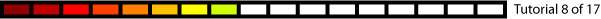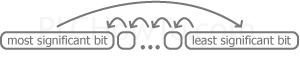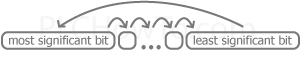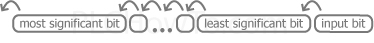# PLC Rotate & ShiftRotate functions rotate bits within an Integer Register.  There are typically 2 options:

• Rotate Left or Right?
• How many bits would you like rotated?

Rotate Left:Example: 8 bit Int with a value 0xC5
11000101 = Value in binary
10001011 = Rotated Left 1 bit
00010111 = Rotated Left 2 bits

Rotate Right:Example: 8 bit Int with a value 0xC5
11000101 = Value in binary
11100010 = Rotated Right 1 bit
01110001 = Rotated Right 2 bits

Shift works just like Rotate, except for what happens to the end bits.  On one side the bits are shifted out and the other bits are shifted in.  There are typically 3 options:

• Shift Left or Right?
• How many bits would you like shifted?
• What value would you like shifted in?  This can be a fixed value (0 or 1) or may come from a register.
Shift Left:Example: 8 bit Int with a value 0xC5
11000101 = Value in binary
10001011 = Shifted Left 1 bit  (Shifting in value 1)
00010111 = Shifted Left 2 bits  (Shifting in value 1)

Shift Right:Example: 8 bit Int with a value 0xC5
11000101 = Value in binary
01100010 = Shifted Right 1 bit  (Shifting in value 0)
00110001 = Shifted Right 2 bits  (Shifting in value 0)